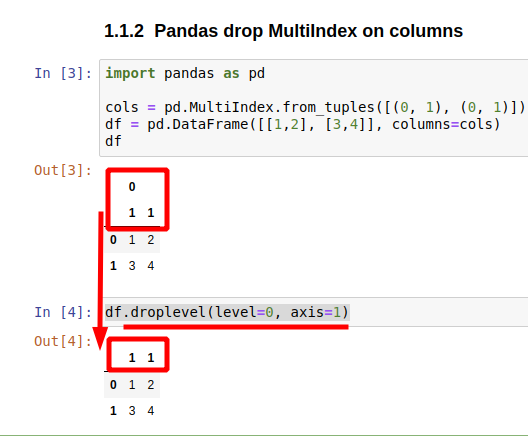Here are several approaches to drop levels of MultiIndex in a Pandas DataFrame:

• `droplevel` - completely drop MultiIndex level
• `reset_index` - remove levels of MultiIndex while storing data into columns/rows

If you want to find more about: What is a DataFrame MultiIndex in Pandas

## Step 1: Pandas drop MultiIndex by method - droplevel

### Pandas drop MultiIndex on index/rows

Method `droplevel()` will remove one, several or all levels from a MultiIndex. Let's check the default execution by next example:

``````import pandas as pd

cols = pd.MultiIndex.from_tuples([(0, 1), (0, 1)])
df = pd.DataFrame([[1,2], [3,4]], index=cols)
df
``````
0 1
0 1 1 2
1 3 4

We can drop level 0 of the MultiIndex by:

``````df.droplevel(level=0)
``````

which will result in:

0 1
1 1 2
1 3 4

or level 1:

``````df.droplevel(level=1)
``````

### Pandas drop MultiIndex on columns

If the hierarchical indexing is on the columns then we can drop levels by parameter `axis`:

``````df.droplevel(level=0, axis=1)
``````

### Get column names of the dropped columns

If you like to get the names of the columns which will be dropped you can use next syntax:

• for columns:
``````df.columns.droplevel(level=0)
``````
• for rows:
``````df.index.droplevel(level=0)
``````

which will result of single index:

Int64Index([1, 1], dtype='int64')

### Pandas drop MultiIndex with `.columns.droplevel(level=0)`

So we can drop level of MultiIndex with a simple reset of the column names like:

``````df.columns = df.columns.droplevel(level=0)
``````## Step 2: Pandas drop MultiIndex to column values by reset_index

### Drop all levels of MultiIndex to columns

Use reset_index if you like to drop the MultiIndex while keeping the information from it. Let's do a quick demo:

``````import pandas as pd

cols = pd.MultiIndex.from_tuples([(0, 1), (0, 1)])
df = pd.DataFrame([[1,2], [3,4]], index=cols)
``````
0 1
0 1 1 2
1 3 4

After reset of the MultiIndex we will get:

``````df.reset_index()
``````
level_0 level_1 0 1
0 0 1 1 2
1 0 1 3 4

### Reset single level of MultiIndex

``````df.reset_index(level=1)
``````

## Typical Errors on Pandas Drop MultiIndex

When the droplevel is invoked on wrong axis: columns or rows like:

``````cols = pd.MultiIndex.from_tuples([(0, 1), (0, 1)])
df = pd.DataFrame([[1,2], [3,4]], index=cols)

df.columns.droplevel()
``````

error is raised:

ValueError: Cannot remove 1 levels from an index with 1 levels: at least one level must be left.

Another example is when the levels are less the one which should be dropped:

``````cols = pd.MultiIndex.from_tuples([(0, 1), (0, 1)])
df = pd.DataFrame([[1,2], [3,4]], index=cols)

df.columns.droplevel(level=3)
``````

IndexError: Too many levels: Index has only 1 level, not 4